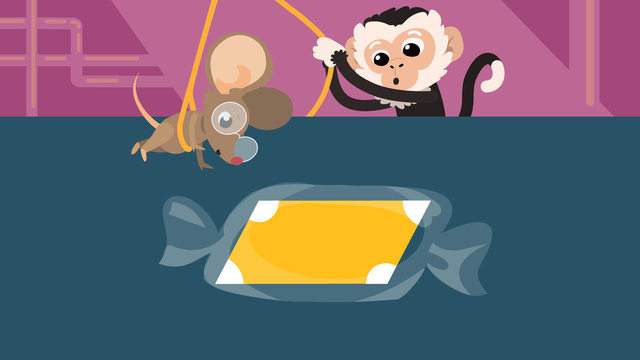Rating

Ø 5.0 / 1 ratings

The authorsTeam Digital
CCSS.MATH.CONTENT.3.G.A.1

## Basics on the topicQuadrilaterals (Rhombus, Parallelogram, Trapezoid)

### Content

Nico and Nia have snuck into a candy factory, where they set off a machine. Candy comes down a machine in different shapes, and they need to sort these into the correct boxes to avoid trouble! They learn about the rhombus shape, parallelogram shape, and trapezoid shape. This means they are able to avoid trouble because they now know the characteristics of a rhombus, the characteristics of a parallelogram, and the characteristics of a trapezoid!### What is a Parallelogram Shape?

Parallelogram definition geometry: A parallelogram is a quadrilateral with two pairs of parallel sides.

#### Parallelogram Properties

The properties of a parallelogram are:

• Opposite angles are equal
• Two pairs of parallel sides

The following illustration shows a parallelogram example with properties.• What does a parallelogram look like? A parallelogram is a quadrilateral with opposite angles that are equal, and two pairs of parallel sides.
• How many sides does a parallelogram have? A parallelogram is a quadrilateral, which means it has four sides.
• Does a parallelogram have four right angles? A parallelogram CAN have four right angles, as long as all the properties of a parallelogram are met.

### What is a Rhombus Shape?

Rhombus definition geometry: A rhombus is a quadrilateral with all sides equal in length.

#### Rhombus Properties

The properties of a rhombus are:

• Opposite angles are equal
• Four sides are ALL equal in length
• Two pairs of parallel sides

The following illustration shows a rhombus example with properties.• Is a rhombus a parallelogram? A rhombus is a parallelogram because it has two pairs of opposite sides.
• How many sides does a rhombus have? A rhombus has four sides.
• Is a rhombus a quadrilateral? A rhombus is a quadrilateral because it has four sides.
• Does a rhombus have right angles? A rhombus CAN have right angles, but it doesn’t always need to have a right angle to be a rhombus.

### What is a Trapezoid Shape?

Trapezoid definition geometry: A trapezoid is a quadrilateral with one pair of parallel sides.

#### Trapezoid Properties

The attributes of a trapezoid are:

• One pair of parallel sides

The following illustration shows a trapezoid example with properties.